# Arithmetic - math word problems

1. Two friends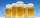Two friends met as a good man perish together for a beer. After recovery the most important topics (politics, women, football ...), one asks: - And how many do you have children? - I have 3 children. - And how many years have? Friend already not want to a
2. Company accountThe company had an account of EUR 10,000. On Monday it withdraw 3,000 euros and Tuesday 6,000 euros. How much did the euro remain in the account?
3. Star equationWrite digits instead of stars so that the sum of the written digits is odd and is true equality: 42 · ∗8 = 2 ∗∗∗
4. Christmas trees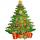Salesman sold Christmas trees: spruce for € 22, pine for € 25 and fir for € 33. At the morning he had the same number of spruce, fir and pine. At the evening he had all the trees entirely sold for € 3,600. How many trees that day salesman sold?
5. Four families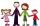Four families were on a joint trip. In the first family, there were three siblings, namely Alica, Betka and Cyril. In the second family were four siblings, namely David, Erik, Filip and Gabika. In the third family, there were two siblings, namely Hugo an
6. DiagonalsGiven a rhombus ABCD with a diagonalsl length of 8 cm and 12 cm. Calculate the side length and content of the rhombus.
7. David numberJana and David train the addition of the decimal numbers so that each of them will write a single number and these two numbers then add up. The last example was 11.11. David's number had the same number of digits before the decimal point, the Jane's numbe
8. DivisionWhich number in division 16 give 12 and the rest 3?
9. Swallows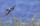7 swallows 8 tits and 8 sparrows sitting on a wire. How many total flyoff bird sitting on a wire?
10. Unknown number 16My number's tens is three times more then ones My number's ones is twice the number of thousands and my number's hundreds is half the number of of tens. I have two ones. which number am I?
11. Flowers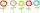The flower has six flowers and each flower has a number. These are the numbers: 20,40,39,28,8,9. What number will be in the middle of the flower so that the numbers come from the flowers when we subtract and add?Grandfather have three barrels each with a capacity 2HL in the garden. How many times he can fill 10L can if two barrels are full and the third is filled with water to the half? How long it lasts water when irrigate the garden 125 liters a day?
13. Car parking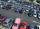The car park has 80 cars when 53 cars leaves. How many car are there?
14. Summands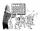Find two summands of the number 42, so that its product is minimized.
15. Octahedron - sumOn each wall of a regular octahedron is written one of the numbers 1, 2, 3, 4, 5, 6, 7 and 8, wherein on different sides are different numbers. For each wall John make the sum of the numbers written of three adjacent walls. Thus got eight sums, which also
16. Unit rateFind unit rate: 6,840 customers in 45 days
17. Three vectorsThe three forces whose amplitudes are in ratio 9:10:17 act in the plane at one point so that they are in balance. Determine the angles of the each two forces.
18. ForcesForces with magnitudes F1 = 42N and F2 = 35N act at a common point and make an angle of 77°12'. How big is their resultant?
19. Value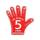Find the value of the expression: 6!·10^-3
20. Book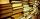Jitka read on holidays book that has 180 pages. In the first week read 45 pages. In the second week she read 15 pages more than the first week. How many pages left to read it yet?

Do you have an interesting mathematical word problem that you can't solve it? Submit math problem, and we can try to solve it.

We will send a solution to your e-mail address. Solved examples are also published here. Please enter the e-mail correctly and check whether you don't have a full mailbox.

Please do not submit problems from current active competitions such as Mathematical Olympiad, correspondence seminars etc...